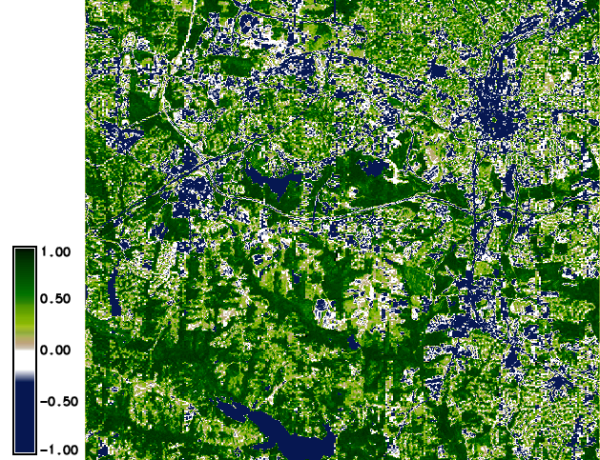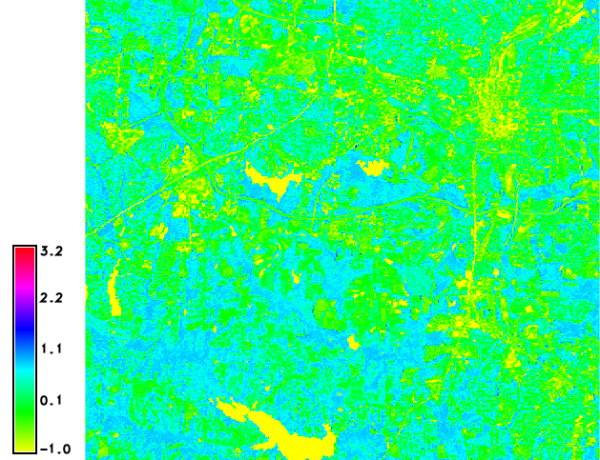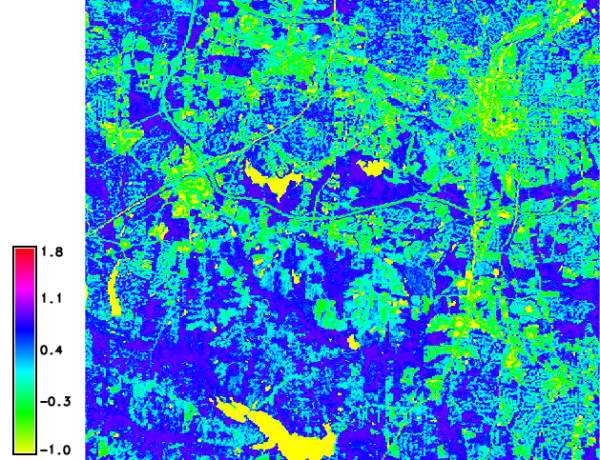Note: This document is for an older version of GRASS GIS that will be discontinued soon. You should upgrade, and read the current manual page.

## NAME

i.vi - Calculates different types of vegetation indices.
Uses red and nir bands mostly, and some indices require additional bands.

## KEYWORDS

imagery, vegetation index, biophysical parameters, NDVI

## SYNOPSIS

i.vi
i.vi --help
i.vi output=name viname=type [red=name] [nir=name] [green=name] [blue=name] [band5=name] [band7=name] [soil_line_slope=float] [soil_line_intercept=float] [soil_noise_reduction=float] [storage_bit=integer] [--overwrite] [--help] [--verbose] [--quiet] [--ui]

### Flags:

--overwrite
Allow output files to overwrite existing files
--help
Print usage summary
--verbose
Verbose module output
--quiet
Quiet module output
--ui
Force launching GUI dialog

### Parameters:

output=name [required]
Name for output raster map
viname=type [required]
Type of vegetation index
Options: arvi, dvi, evi, evi2, gvi, gari, gemi, ipvi, msavi, msavi2, ndvi, ndwi, pvi, savi, sr, vari, wdvi
Default: ndvi
arvi: Atmospherically Resistant Vegetation Index
dvi: Difference Vegetation Index
evi: Enhanced Vegetation Index
evi2: Enhanced Vegetation Index 2
gvi: Green Vegetation Index
gari: Green Atmospherically Resistant Vegetation Index
gemi: Global Environmental Monitoring Index
ipvi: Infrared Percentage Vegetation Index
msavi: Modified Soil Adjusted Vegetation Index
msavi2: second Modified Soil Adjusted Vegetation Index
ndvi: Normalized Difference Vegetation Index
ndwi: Normalized Difference Water Index
pvi: Perpendicular Vegetation Index
sr: Simple Ratio
vari: Visible Atmospherically Resistant Index
wdvi: Weighted Difference Vegetation Index
red=name
Name of input red channel surface reflectance map
Range: [0.0;1.0]
nir=name
Name of input nir channel surface reflectance map
Range: [0.0;1.0]
green=name
Name of input green channel surface reflectance map
Range: [0.0;1.0]
blue=name
Name of input blue channel surface reflectance map
Range: [0.0;1.0]
band5=name
Name of input 5th channel surface reflectance map
Range: [0.0;1.0]
band7=name
Name of input 7th channel surface reflectance map
Range: [0.0;1.0]
soil_line_slope=float
Value of the slope of the soil line (MSAVI only)
soil_line_intercept=float
Value of the intercept of the soil line (MSAVI only)
soil_noise_reduction=float
Value of the factor of reduction of soil noise (MSAVI only)
storage_bit=integer
Maximum bits for digital numbers
If data is in Digital Numbers (i.e. integer type), give the max bits (i.e. 8 for Landsat -> [0-255])
Options: 7, 8, 10, 16
Default: 8

## DESCRIPTION

i.vi calculates vegetation indices based on biophysical parameters.
• ARVI: atmospherically resistant vegetation indices
• DVI: Difference Vegetation Index
• EVI: Enhanced Vegetation Index
• EVI2: Enhanced Vegetation Index 2
• GARI: Green atmospherically resistant vegetation index
• GEMI: Global Environmental Monitoring Index
• GVI: Green Vegetation Index
• IPVI: Infrared Percentage Vegetation Index
• MSAVI2: second Modified Soil Adjusted Vegetation Index
• MSAVI: Modified Soil Adjusted Vegetation Index
• NDVI: Normalized Difference Vegetation Index
• NDWI: Normalized Difference Water Index
• PVI: Perpendicular Vegetation Index
• RVI: ratio vegetation index
• SAVI: Soil Adjusted Vegetation Index
• SR: Simple Vegetation ratio
• WDVI: Weighted Difference Vegetation Index

### Background for users new to remote sensing

Vegetation Indices are often considered the entry point of remote sensing for Earth land monitoring. They are suffering from their success, in terms that often people tend to harvest satellite images from online sources and use them directly in this module.

Satellite imagery is commonly stored in Digital Number (DN) for storage purposes; e.g., Landsat5 data is stored in 8bit values (ranging from 0 to 255), other satellites maybe stored in 10 or 16 bits. If the data is provided in DN, this implies that this imagery is "uncorrected". What this means is that the image is what the satellite sees at its position and altitude in space (stored in DN). This is not the signal at ground yet. We call this data at-satellite or at-sensor. Encoded in the 8bits (or more) is the amount of energy sensed by the sensor inside the satellite platform. This energy is called radiance-at-sensor. Generally, satellites image providers encode the radiance-at-sensor into 8bit (or more) through an affine transform equation (y=ax+b). In case of using Landsat imagery, look at the i.landsat.toar for an easy way to transform DN to radiance-at-sensor. If using Aster data, try the i.aster.toar module.

Finally, once having obtained the radiance at sensor values, still the atmosphere is between sensor and Earth's surface. This fact needs to be corrected to account for the atmospheric interaction with the sun energy that the vegetation reflects back into space. This can be done in two ways for Landsat. The simple way is through i.landsat.toar, use e.g. the DOS correction. The more accurate way is by using i.atcorr (which works for many satellite sensors). Once the atmospheric correction has been applied to the satellite data, data vales are called surface reflectance. Surface reflectance is ranging from 0.0 to 1.0 theoretically (and absolutely). This level of data correction is the proper level of correction to use with i.vi.

### Vegetation Indices

ARVI: Atmospheric Resistant Vegetation Index

ARVI is resistant to atmospheric effects (in comparison to the NDVI) and is accomplished by a self correcting process for the atmospheric effect in the red channel, using the difference in the radiance between the blue and the red channels (Kaufman and Tanre 1996).

```arvi( redchan, nirchan, bluechan )

ARVI = (nirchan - (2.0*redchan - bluechan)) /
( nirchan + (2.0*redchan - bluechan))
```

DVI: Difference Vegetation Index

```dvi( redchan, nirchan )

DVI = ( nirchan - redchan )
```

EVI: Enhanced Vegetation Index

The enhanced vegetation index (EVI) is an optimized index designed to enhance the vegetation signal with improved sensitivity in high biomass regions and improved vegetation monitoring through a de-coupling of the canopy background signal and a reduction in atmosphere influences (Huete A.R., Liu H.Q., Batchily K., van Leeuwen W. (1997). A comparison of vegetation indices global set of TM images for EOS-MODIS. Remote Sensing of Environment, 59:440-451).

```evi( bluechan, redchan, nirchan )

EVI = 2.5 * ( nirchan - redchan ) /
( nirchan + 6.0 * redchan - 7.5 * bluechan + 1.0 )
```

EVI2: Enhanced Vegetation Index 2

A 2-band EVI (EVI2), without a blue band, which has the best similarity with the 3-band EVI, particularly when atmospheric effects are insignificant and data quality is good (Zhangyan Jiang ; Alfredo R. Huete ; Youngwook Kim and Kamel Didan 2-band enhanced vegetation index without a blue band and its application to AVHRR data. Proc. SPIE 6679, Remote Sensing and Modeling of Ecosystems for Sustainability IV, 667905 (october 09, 2007) doi:10.1117/12.734933).

```evi2( redchan, nirchan )

EVI2 = 2.5 * ( nirchan - redchan ) /
( nirchan + 2.4 * redchan + 1.0 )
```

GARI: green atmospherically resistant vegetation index

The formula was actually defined: Gitelson, Anatoly A.; Kaufman, Yoram J.; Merzlyak, Mark N. (1996) Use of a green channel in remote sensing of global vegetation from EOS- MODIS, Remote Sensing of Environment 58 (3), 289-298. doi:10.1016/s0034-4257(96)00072-7

```gari( redchan, nirchan, bluechan, greenchan )

GARI = ( nirchan - (greenchan - (bluechan - redchan))) /
( nirchan + (greenchan - (bluechan - redchan)))
```

GEMI: Global Environmental Monitoring Index

```gemi( redchan, nirchan )

GEMI = (( (2*((nirchan * nirchan)-(redchan * redchan)) +
1.5*nirchan+0.5*redchan) / (nirchan + redchan + 0.5)) *
(1 - 0.25 * (2*((nirchan * nirchan)-(redchan * redchan)) +
1.5*nirchan+0.5*redchan) / (nirchan + redchan + 0.5))) -
( (redchan - 0.125) / (1 - redchan))
```

GVI: Green Vegetation Index

```gvi( bluechan, greenchan, redchan, nirchan, chan5chan, chan7chan)

GVI = ( -0.2848 * bluechan - 0.2435 * greenchan -
0.5436 * redchan + 0.7243 * nirchan + 0.0840 * chan5chan-
0.1800 * chan7chan)
```

IPVI: Infrared Percentage Vegetation Index

```ipvi( redchan, nirchan )

IPVI = nirchan/(nirchan+redchan)
```

MSAVI2: second Modified Soil Adjusted Vegetation Index

```msavi2( redchan, nirchan )

MSAVI2 = (1/2)*(2*NIR+1-sqrt((2*NIR+1)^2-8*(NIR-red)))
```

MSAVI: Modified Soil Adjusted Vegetation Index

```msavi( redchan, nirchan )

MSAVI = s(NIR-s*red-a) / (a*NIR+red-a*s+X*(1+s*s))
```
where a is the soil line intercept, s is the soil line slope, and X is an adjustment factor which is set to minimize soil noise (0.08 in original papers).

NDVI: Normalized Difference Vegetation Index

```ndvi( redchan, nirchan )

Satellite specific band numbers ([NIR, Red]):
MSS Bands        = [ 7,  5]
TM1-5,7 Bands    = [ 4,  3]
TM8 Bands        = [ 5,  4]
Sentinel-2 Bands = [ 8,  4]
AVHRR Bands      = [ 2,  1]
SPOT XS Bands    = [ 3,  2]
AVIRIS Bands     = [51, 29]

NDVI = (NIR - Red) / (NIR + Red)
```

NDWI: Normalized Difference Water Index (after McFeeters, 1996)

This index is suitable to detect water bodies.

```ndwi( greenchan, nirchan )

NDWI = (green - NIR) / (green + NIR)
```

The water content of leaves can be estimated with another NDWI (after Gao, 1996):

```ndwi( greenchan, nirchan )

NDWI = (NIR - SWIR) / (NIR + SWIR)
```
This index is important for monitoring vegetation health (not implemented).

PVI: Perpendicular Vegetation Index

```pvi( redchan, nirchan )

PVI = sin(a)NIR-cos(a)red
```
for a isovegetation lines (lines of equal vegetation) would all be parallel to the soil line therefore a=1.

```savi( redchan, nirchan )

SAVI = ((1.0+0.5)*(nirchan - redchan)) / (nirchan + redchan +0.5)
```

SR: Simple Vegetation ratio

```sr( redchan, nirchan )

SR = (nirchan/redchan)
```

VARI: Visible Atmospherically Resistant Index VARI was designed to introduce an atmospheric self-correction (Gitelson A.A., Kaufman Y.J., Stark R., Rundquist D., 2002. Novel algorithms for estimation of vegetation fraction Remote Sensing of Environment (80), pp76-87.)

```vari = ( bluechan, greenchan, redchan )

VARI = (green - red ) / (green + red - blue)
```

WDVI: Weighted Difference Vegetation Index

```wdvi( redchan, nirchan, soil_line_weight )

WDVI = nirchan - a * redchan
if(soil_weight_line == None):
a = 1.0   #slope of soil line
```

## EXAMPLES

### Calculation of DVI

The calculation of DVI from the reflectance values is done as follows:
```g.region raster=band.1 -p
i.vi blue=band.1 red=band.3 nir=band.4 viname=dvi output=dvi
r.univar -e dvi
```

### Calculation of EVI

The calculation of EVI from the reflectance values is done as follows:
```g.region raster=band.1 -p
i.vi blue=band.1 red=band.3 nir=band.4 viname=evi output=evi
r.univar -e evi
```

### Calculation of EVI2

The calculation of EVI2 from the reflectance values is done as follows:
```g.region raster=band.3 -p
i.vi red=band.3 nir=band.4 viname=evi2 output=evi2
r.univar -e evi2
```

### Calculation of GARI

The calculation of GARI from the reflectance values is done as follows:
```g.region raster=band.1 -p
i.vi blue=band.1 green=band.2 red=band.3 nir=band.4 viname=gari output=gari
r.univar -e gari
```

### Calculation of GEMI

The calculation of GEMI from the reflectance values is done as follows:
```g.region raster=band.3 -p
i.vi red=band.3 nir=band.4 viname=gemi output=gemi
r.univar -e gemi
```

### Calculation of GVI

The calculation of GVI (Green Vegetation Index - Tasseled Cap) from the reflectance values is done as follows:
```g.region raster=band.3 -p
# assuming Landsat-7
i.vi blue=band.1 green=band.2 red=band.3 nir=band.4 band5=band.5 band7=band.7 viname=gvi output=gvi
r.univar -e gvi
```

### Calculation of IPVI

The calculation of IPVI from the reflectance values is done as follows:
```g.region raster=band.3 -p
i.vi red=band.3 nir=band.4 viname=ipvi output=ipvi
r.univar -e ipvi
```

### Calculation of MSAVI

The calculation of MSAVI from the reflectance values is done as follows:
```g.region raster=band.3 -p
i.vi red=band.3 nir=band.4 viname=msavi output=msavi
r.univar -e msavi
```

### Calculation of NDVI

The calculation of NDVI from the reflectance values is done as follows:
```g.region raster=band.3 -p
i.vi red=band.3 nir=band.4 viname=ndvi output=ndvi
r.univar -e ndvi
```

### Calculation of NDWI

The calculation of NDWI from the reflectance values is done as follows:
```g.region raster=band.2 -p
i.vi green=band.2 nir=band.4 viname=ndwi output=ndwi
r.colors ndwi color=byg -n
r.univar -e ndwi
```

### Calculation of PVI

The calculation of PVI from the reflectance values is done as follows:
```g.region raster=band.3 -p
i.vi red=band.3 nir=band.4 viname=pvi output=pvi
r.univar -e pvi
```

### Calculation of SAVI

The calculation of SAVI from the reflectance values is done as follows:
```g.region raster=band.3 -p
i.vi red=band.3 nir=band.4 viname=savi output=savi
r.univar -e savi
```

### Calculation of SR

The calculation of SR from the reflectance values is done as follows:
```g.region raster=band.3 -p
i.vi red=band.3 nir=band.4 viname=sr output=sr
r.univar -e sr
```

### Calculation of VARI

The calculation of VARI from the reflectance values is done as follows:
```g.region raster=band.3 -p
i.vi blue=band.2 green=band.3 red=band.4 viname=vari output=vari
r.univar -e vari
```

### Landsat TM7 example

The following examples are based on a LANDSAT TM7 scene included in the North Carolina sample dataset.

#### Preparation: DN to reflectance

As a first step, the original DN (digital number) pixel values must be converted to reflectance using i.landsat.toar. To do so, we make a copy (or rename the channels) to match i.landsat.toar's input scheme:

```g.copy raster=lsat7_2002_10,lsat7_2002.1
g.copy raster=lsat7_2002_20,lsat7_2002.2
g.copy raster=lsat7_2002_30,lsat7_2002.3
g.copy raster=lsat7_2002_40,lsat7_2002.4
g.copy raster=lsat7_2002_50,lsat7_2002.5
g.copy raster=lsat7_2002_61,lsat7_2002.61
g.copy raster=lsat7_2002_62,lsat7_2002.62
g.copy raster=lsat7_2002_70,lsat7_2002.7
g.copy raster=lsat7_2002_80,lsat7_2002.8
```

Calculation of reflectance values from DN using DOS1 (metadata obtained from p016r035_7x20020524.met.gz):

```i.landsat.toar input=lsat7_2002. output=lsat7_2002_toar. sensor=tm7 \
method=dos1 date=2002-05-24 sun_elevation=64.7730999 \
product_date=2004-02-12 gain=HHHLHLHHL
```
The resulting Landsat channels are names lsat7_2002_toar.1 .. lsat7_2002_toar.8.

#### Calculation of NDVI

The calculation of NDVI from the reflectance values is done as follows:
```g.region raster=lsat7_2002_toar.3 -p
i.vi red=lsat7_2002_toar.3 nir=lsat7_2002_toar.4 viname=ndvi \
output=lsat7_2002.ndvi
r.colors lsat7_2002.ndvi color=ndvi

d.mon wx0
d.rast.leg lsat7_2002.ndvi
```North Carolina dataset: NDVI

#### Calculation of ARVI

The calculation of ARVI from the reflectance values is done as follows:
```g.region raster=lsat7_2002_toar.3 -p
i.vi blue=lsat7_2002_toar.1 red=lsat7_2002_toar.3 nir=lsat7_2002_toar.4 \
viname=arvi output=lsat7_2002.arvi

d.mon wx0
d.rast.leg lsat7_2002.arvi
```North Carolina dataset: ARVI

#### Calculation of GARI

The calculation of GARI from the reflectance values is done as follows:
```g.region raster=lsat7_2002_toar.3 -p
i.vi blue=lsat7_2002_toar.1 green=lsat7_2002_toar.2 red=lsat7_2002_toar.3 \
nir=lsat7_2002_toar.4 viname=gari output=lsat7_2002.gari

d.mon wx0
d.rast.leg lsat7_2002.gari
```North Carolina dataset: GARI

## NOTES

Originally from kepler.gps.caltech.edu (FAQ):

A FAQ on Vegetation in Remote Sensing
Written by Terrill W. Ray, Div. of Geological and Planetary Sciences, California Institute of Technology, email: terrill@mars1.gps.caltech.edu

Snail Mail: Terrill Ray
Division of Geological and Planetary Sciences
Caltech, Mail Code 170-25

i.albedo, i.aster.toar, i.landsat.toar, i.atcorr, i.tasscap

## REFERENCES

AVHRR, Landsat TM5:
• Bastiaanssen, W.G.M., 1995. Regionalization of surface flux densities and moisture indicators in composite terrain; a remote sensing approach under clear skies in mediterranean climates. PhD thesis, Wageningen Agricultural Univ., The Netherland, 271 pp. (PDF)
• Index DataBase: List of available Indices

## AUTHORS

Baburao Kamble, Asian Institute of Technology, Thailand
Yann Chemin, Asian Institute of Technology, Thailand

## SOURCE CODE

Available at: i.vi source code (history)

Latest change: Sunday Nov 28 08:41:15 2021 in commit: 951d8a873c223dafc7496f522f94185142d973db

Note: This document is for an older version of GRASS GIS that will be discontinued soon. You should upgrade, and read the current manual page.

© 2003-2023 GRASS Development Team, GRASS GIS 7.8.8dev Reference Manual